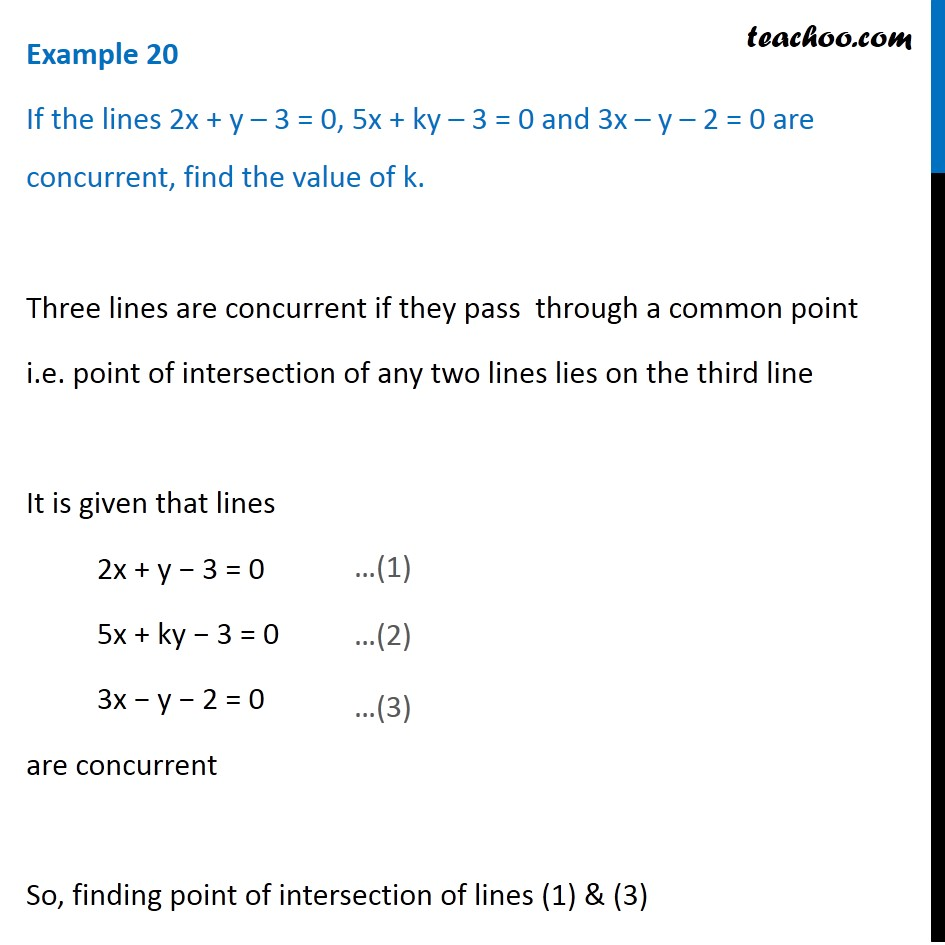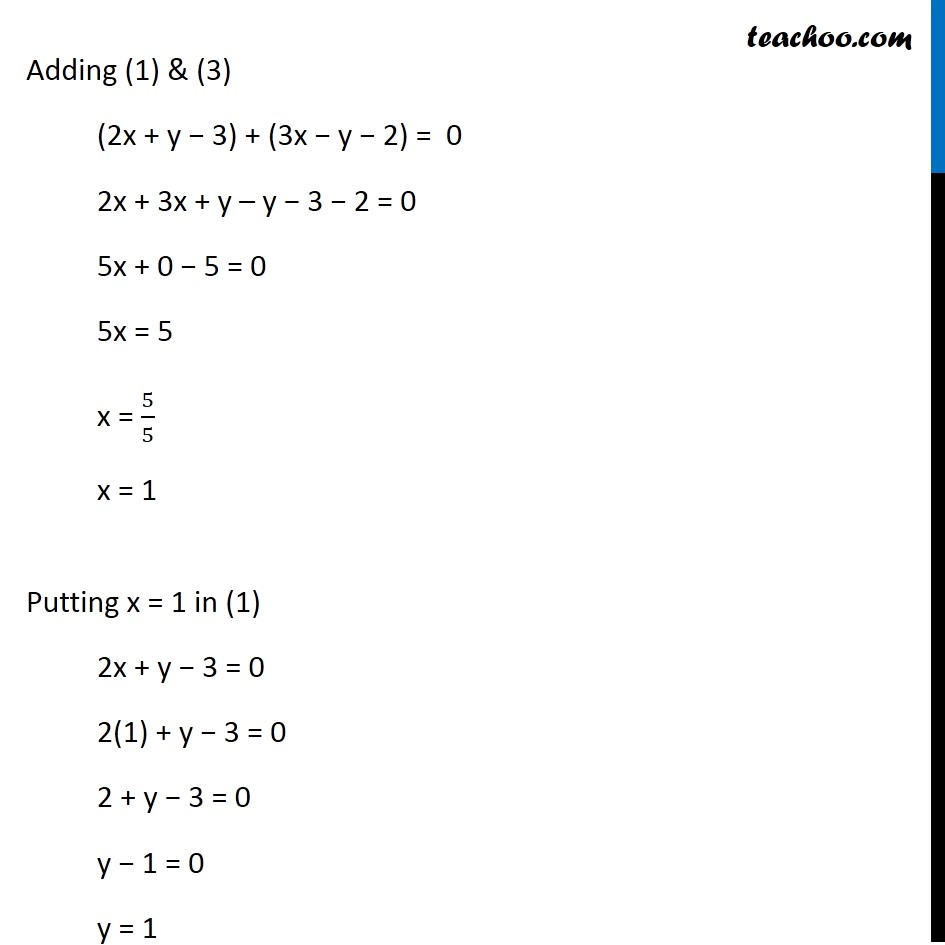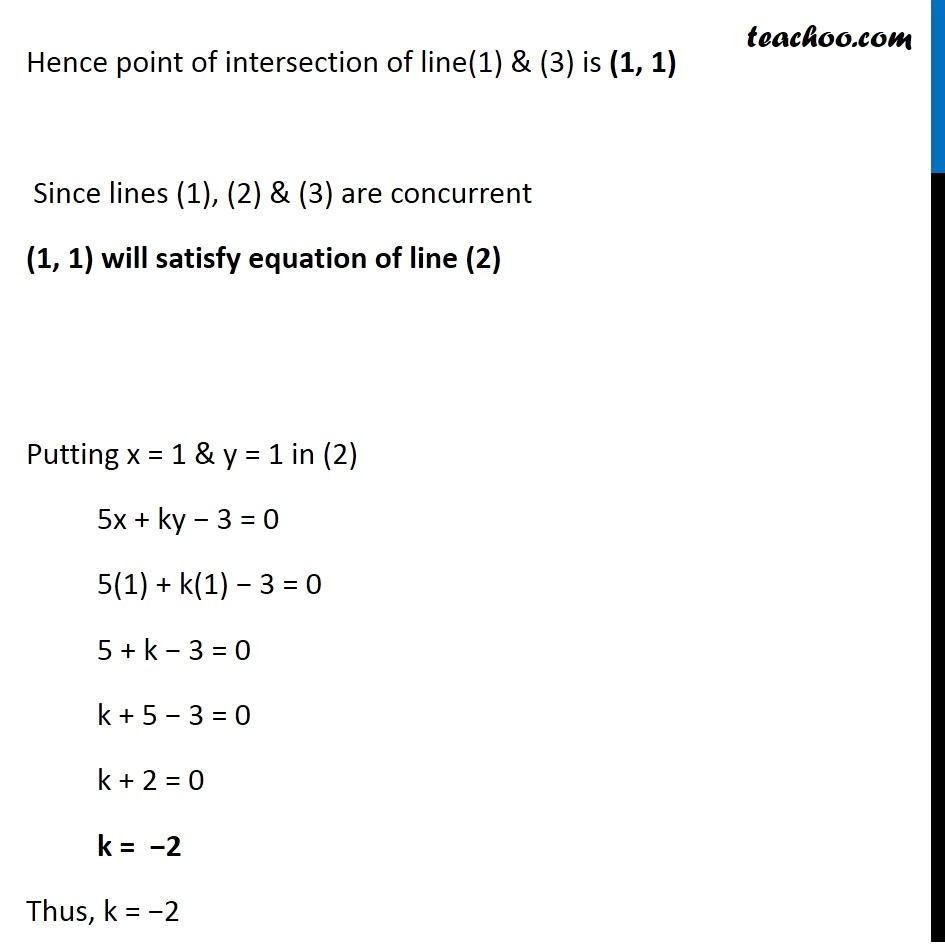Examples

Chapter 9 Class 11 Straight Lines
Serial order wiseLearn in your speed, with individual attention - Teachoo Maths 1-on-1 Class

### Transcript

Example 11 If the lines 2x + y – 3 = 0, 5x + ky – 3 = 0 and 3x – y – 2 = 0 are concurrent, find the value of k. Three lines are concurrent if they pass through a common point i.e. point of intersection of any two lines lies on the third line It is given that lines 2x + y − 3 = 0 5x + ky − 3 = 0 3x − y − 2 = 0 are concurrent So, finding point of intersection of lines (1) & (3) Adding (1) & (3) (2x + y − 3) + (3x − y − 2) = 0 2x + 3x + y – y − 3 − 2 = 0 5x + 0 − 5 = 0 5x = 5 x = 5/5 x = 1 Putting x = 1 in (1) 2x + y − 3 = 0 2(1) + y − 3 = 0 2 + y − 3 = 0 y − 1 = 0 y = 1 Hence point of intersection of line(1) & (3) is (1, 1) Since lines (1), (2) & (3) are concurrent (1, 1) will satisfy equation of line (2) Putting x = 1 & y = 1 in (2) 5x + ky − 3 = 0 5(1) + k(1) − 3 = 0 5 + k − 3 = 0 k + 5 − 3 = 0 k + 2 = 0 k = −2 Thus, k = −2